Next: Ampère's circuital law Up: Time-independent Maxwell equations Previous: Ampère's law

## Magnetic monopoles?

Suppose that we have an infinite straight wire carrying an electric current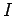. Let the wire be aligned along the-axis. The magnetic field generated by such a wire is written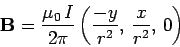(254)

in Cartesian coordinates, where. The divergence of this field is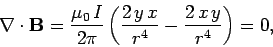(255)

where use has been made of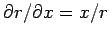, etc. We saw in Sect. 3.4 that the divergence of the electric field appeared, at first sight, to be zero. But, in reality, it was a delta-function, because the volume integral of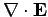was non-zero. Does the same sort of thing happen for the divergence of the magnetic field? Well, if we could find a closed surfacefor whichthen, according to Gauss' theorem,, whereis the volume enclosed by. This would certainly imply that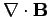is some sort of delta-function. So, can we find such a surface? The short answer is, no. Consider a cylindrical surface aligned with the wire. The magnetic field is everywhere tangential to the outward surface element, so this surface certainly has zero magnetic flux coming out of it. In fact, it is impossible to invent any closed surface for which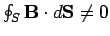withgiven by Eq. (254) (if you do not believe this, try it yourselves!). This suggests that the divergence of a magnetic field generated by steady electric currents really is zero. Admittedly, we have only proved this for infinite straight currents, but, as will be demonstrated presently, it is true in general.

If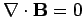thenis a solenoidal vector field. In other words, field-lines ofnever begin or end. This is certainly the case in Eq. (254) where the field-lines are a set of concentric circles centred on the-axis. What about magnetic fields generated by permanent magnets (the modern equivalent of loadstones)? Do they also never begin or end? Well, we know that a conventional bar magnet has both a North and South magnetic pole (like the Earth). If we track the magnetic field-lines with a small compass they all emanate from the South pole, spread out, and eventually reconverge on the North pole (see Fig. 31). It appears likely (but we cannot prove it with a compass) that the field-lines inside the magnet connect from the North to the South pole so as to form closed loops which never begin or end.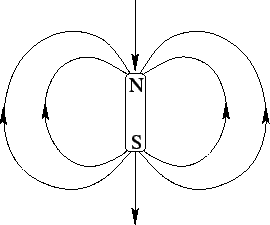Can we produce an isolated North or South magnetic pole: for instance, by snapping a bar magnet in two? A compass needle would always point towards an isolated North pole, so this would act like a negative magnetic charge.'' Likewise, a compass needle would always point away from an isolated South pole, so this would act like a positive magnetic charge.'' It is clear from Fig. 32 that if we take a closed surfacecontaining an isolated magnetic pole, which is usually termed a magnetic monopole, then: the flux will be positive for an isolated South pole, and negative for an isolated North pole. It follows from Gauss' theorem that ifthen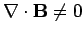. Thus, the statement that magnetic fields are solenoidal, or that, is equivalent to the statement that there are no magnetic monopoles. It is not clear, a priori, that this is a true statement. In fact, it is quite possible to formulate electromagnetism so as to allow for magnetic monopoles. However, as far as we know, there are no magnetic monopoles in the Universe. At least, if there are any then they are all hiding from us! We know that if we try to make a magnetic monopole by snapping a bar magnet in two then we just end up with two smaller bar magnets. If we snap one of these smaller magnets in two then we end up with two even smaller bar magnets. We can continue this process down to the atomic level without ever producing a magnetic monopole. In fact, permanent magnetism is generated by electric currents circulating on the atomic scale, so this type of magnetism is not fundamentally different to the magnetism generated by macroscopic currents.In conclusion, all steady magnetic fields in the Universe are generated by circulating electric currents of some description. Such fields are solenoidal: that is, they never begin or end, and satisfy the field equation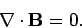(256)

This, incidentally, is the second of Maxwell's equations. Essentially, it says that there is no such thing as a magnetic monopole. We have only proved thatfor steady magnetic fields, but, in fact, this is also the case for time-dependent fields (see later).Next: Ampère's circuital law Up: Time-independent Maxwell equations Previous: Ampère's law
Richard Fitzpatrick 2006-02-02